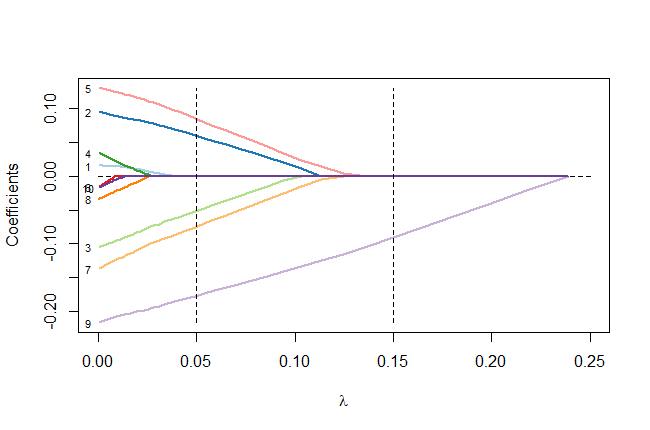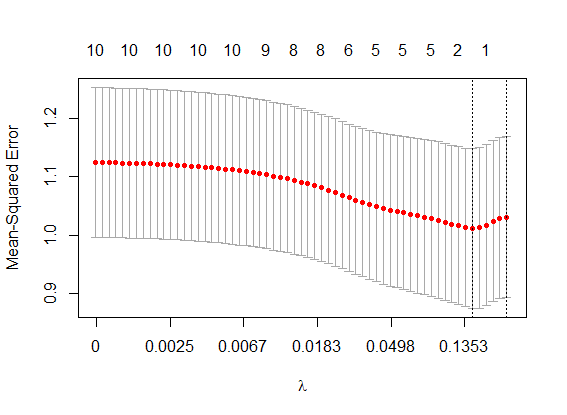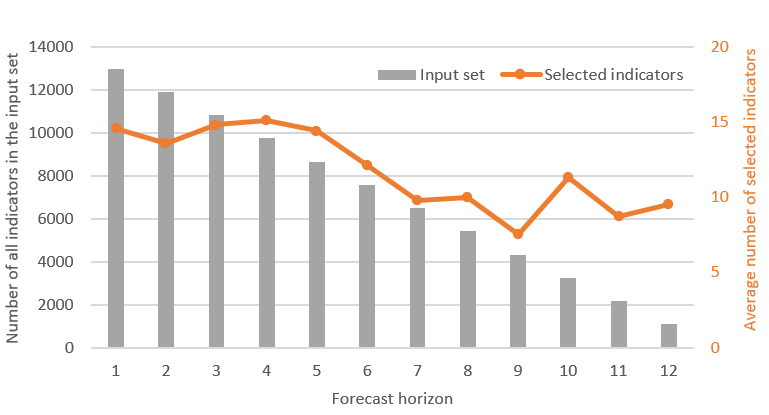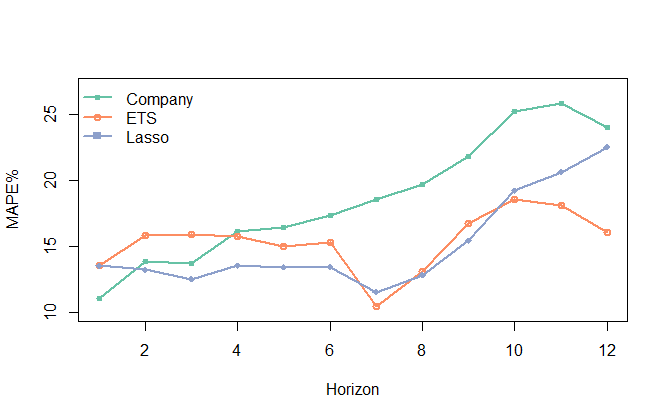By | June 29, 2018

Nikolaos Kourentzes and Yves Sagaert, Foresight: The International Journal of Applied Forecasting, 2018, Issue 48.

This is a modified version of the paper that appears in Foresight issue 48. This provides a simplified version of the modelling methodology described in this paper and applied here and here.

Introduction

Using leading indicators for business forecasting has been relatively rare, partly because our traditional time series methods do not readily allow incorporation of external variables. Nowadays however, we have an abundance of potentially useful indicators, and there is evidence that utilizing relevant ones in a forecasting model can significantly improve forecast accuracy and transparency.

We can define a leading indicator as a numerical variable that contains predictive information for our target variable (e.g., sales) at least as many periods in advance as the forecast lead time. There are several aspects to this definition.

• First, the indicator should be a hard variable; that is, recorded in a useful way for inputting into a statistical model. This means it should have adequate history and be available at the required frequency, after any appropriate transformations (for example, aggregating data into the desired frequency).
• Second, the indicator must contain genuine predictive information, not spurious statistical correlation with the target.
• Third, the indicator must lead the target variable by enough time to be operationally useful. If for example we have a leading indicator that is very informative one month in advance of sales, but we need three-month ahead sales forecast for ordering supplies and materials, this indicator lacks sufficient lead time. (If you are still thinking that it is useful for improving your forecasts, then your forecast lead time is not three but one month – a misjudgment we see all too often!) Note that sometimes it is possible to produce (or source externally) forecasts of indicators, but then poor forecasts here indicators will be damaging! A detailed discussion of the so-called conditional (i.e. using information only up to the time of forecast) and uncoditional forecasting can be found in Principles of Business Forecasting, where alternative forecasting strategies are discussed.

Sources of potential indicators are governmental, banking and private-sector data on macroeconomic, internet search data, social media postings, and an often overlooked source,  the company itself. Strategic, marketing on other company plans, can be useful for constructing leading indicators.

Suppose we have identified a useful leading indicator, how do we use it for forecasting? In the simplest setting, where the target responds linearly to movement in the indicator, we can construct a forecast as Equation (1):$F_{t+h} = M_{t+h} + cX_t$ (1)

where$F_{t+h}$ is the forecast for period t+h, constructed at period t (now!),$M_{t+h}$ represents the time series components – level, trend, and seasonality – of the target at period t+h exclusive of the effect of the leading indicator, and c is the coefficient on a leading indicator$X_t$.  In a regression context,$M_{t+h}$ could comprise a constant, a trend variable, a set of seasonal dummies, or lagged values of the target.

Observe that we use index t for$X_t$, since we require its value to be available at the time we construct the forecast for at least h periods ahead. If the lead were more than h, we would require a forecast for the indicator X. which may introduce more forecast error. Additionally. we might be able to procure forecasts of X from an external source.

Equation (1) can be extended to include many indicators (or lags of the same indicator) in the same fashion as we would include more variables in a multiple regression.

So far, we have assumed that we know a priori which indicators are useful. Realistically, this is not the case – so we need an identification strategy for selecting among potentially numerous potential indicators.

Let’s start by restricting our search to macroeconomic leading indicators, which can readily be sourced from a national statistics bureau. There are thousands, maybe tens of thousands of these that could have predictive information. On top of this, we need to consider various lags of the indicators to identify the most informative lead. For example, is it housing sold three or four months in advance that is most informative for our sales? This can quickly bring the number of potential indicators to several hundreds of thousands.

In fact, the number of potential indicator variables k can be larger than the number of available historical observations in our sample n, which makes it impossible to estimate or evaluate a model and rules out classical regression search strategies, such as stepwise regression.

The lasso regression procedure

A promising solution to this problem comes from the technique called Least Absolute Shrinkage and Selection Operator (Lasso) regression. Lasso was introduced by Robert Tibshirani (1996) and since has become a popular tool in many disciplines. (See Ord et al., 2017, section 9.5.2 for a barebone introduction and Hastie et al., 2015 for a thorough discussion) Lasso regression has the major advantage that it can still select and estimate a model when the number of potential indicator variables is greater than the number of historical observations, k>n.

To estimate the model coefficients in standard regression we minimise the Sum of Squared Error (SSE):$SSE=\sum_{t+1}^{n}{(A_t-F_t)^2}$ (2)

where$A_t$ is the actual observation at time t and$F_t$ is the forecast.  Suppose that the forecast is made up by a constant and k explanatory variables$X_{k,t}$. For the sake of simplicity, we will drop any lags from the notation, and consider any lagged input as different inputs X. Given that$F_t=c_o+\sum_{i=1}^k{c_iX_{i,t}}$, (2) becomes:$SSE=\sum_{t=1}^n{(A_t-c_0-\sum_{i=1}^k{c_iX_{i,t}})^2}$, (3)

where$c_o$ is the constant and$c_i$ the coefficient on indicator$X_{i,t}$.

Equation (3) is called the cost function or loss function of the regression. The regression method finds the values for$c_o$ and$c_i$ that minimize the cost function. When a coefficient is insignificantly different from zero, we typically remove that variable from the regression, achieving variable selection.

If k>n, there is no unique solution for the c coefficients, which renders the model useless. This is because we allow full flexibility to the coefficients, which can take on any values. To overcome the problem, we need to impose restrictions on the coefficients. Lasso does so by modifying Equation (3) so that it includes a penalty for model complexity, as measured by the number of regressors in the model with non-zero coefficients. If we think of Equation (3) as “fitting error” we can conceptually write

Error = (Fitting Error) + λ(Penalty). (4)

The reader may realise that Equation (4) appears quite often in time series modelling. Information criteria, such as Akaike’s Information Criterion (AIC) have a similar structure, although a very different underlying logic and derivation. Information criteria seek to mitigate potential overfitting of a model, by imposing penalties/restrictions to the model fit.

In Lasso, penalty is$\sum_{i=1}^{k}{|c'_i|}$, where$c'_i$ is the coefficient of the standardised variable$X_i$. The intuition behind this penalty is the following: the more non-zero coefficients (i.e. included variables) the bigger the penalty becomes. Therefore, for equation (4) to be minimised, the penalty must become small as well, which forces variables out of the model. Standardization puts all variables on the same scale, preventing larger-valued variables from dominating just because of their scale rather than their importance in explaining the variation of the target variable.

We can now write the cost function of Lasso as:$\sum_{t=1}^{n}{(A'_t - \sum_{i=1}^{k}{c'_i X'_{i,t}})^2} + \lambda \sum_{i=1}^{k}{|c'_i|}$, (5)

where both actuals and input variables have been standardised, denoted by the prime indicator (‘).

Determining λ

Lasso attempts to minimise Equation (5). If$\lambda = 0$ Equation (5) reduces to Equation (3), the conventional regression solution. As λ increases above zero, the penalty becomes increasingly dominant, which pushes the c coefficients toward zero (hence the name “shrinkage”). Those variables with zero coefficients will be removed from the model. Intuitively, it is the coefficients on the least important variables that are first pushed to zero, leaving the more important variables in the model to be estimated.  (“Important” does not mean causal, but merely that it explains some of the variance of the historical data.)

So Lasso will select variables— those that remain— and estimate the values of their coefficients. This is true even when k>n.

A “side-effect” of Lasso regression is that we no longer receive statistical-significance values, as we do with conventional regression.  This is so because Equation (5) violates important assumptions underlying the regression model.

Figure 1 illustrates how the coefficients of ten variables – each distinguished by number and color –shrink as  increases (from left to right), until eventually all are removed from the model.

The coefficient values on the very left of the plot are almost identical to the standard regression solution (λ approximatelly zero). This changes as λ increases. For example, imagine a vertical line at λ=0.05. It’s intersection with the lines in the plot reveal the coefficients’ values (on the vertical axis). Some are already equal to zero: those numbered Similarly, if we look at the coefficients for λ=0.15, only one coefficient (number 9) remains non-zero.Figure 1: Evolution of coefficients for different values of λ. The horizontal lower axis provides the value of λ, where on the left side it starts with λ≈0 and ends with a sufficiently large value to remove all variables. The vertical axis indicates the value of the coefficients. Each variable coefficient is assigned a different colour and are named from 1 to 10.

So the choice of value for λ is very important for the performance of Lasso. But how is λ determined?  The standard procedure is through cross-validation: we separate the historical sample into various subsamples, calculating the cross-validated mean squared error across all subsamples. We then look for λ values that minimises this error. Actually, we often chose a slightly larger λ to avoid overfitting to the cross-validation itself. Figure 2 visualises the result of this process. Here, we ran Lasso regressions for various values of λ – plotted on the horizontal axis – and recorded the cross-validated errors. The right-most vertical line indicates the selected (larger) λ, while the vertical line to its left indicates the minimum cross-validated error.

With λ determined, Lasso proceeds to estimate the coefficients of the remaining variables and at the same time drop any variables accordingly.Figure 2: Cross-validated Mean Squared Error (MSE; red dots) for various values of λ with standard errors (vertical bars) of MSE. Upper horizontal axis indicates the number of non-zero variables. Lower horizontal axis increases at a logarithmic scale.

A case study

Sagaert et al. (2018) showed how Lasso was applied to identify macroeconomic leading indicators for forecasting a tire manufacturer’s sales at a regional level. More specifically, our focus was on forecasting monthly sales for the raw materials in two types of end products for the regions of EU and the US.

The current company practice uses Holt-Winters exponential smoothing to forecast long term sales based on estimates of the trend and seasonal patterns. Management believed however that there are key economic activity indicators that could yield predictive information. For instance, net transported goods can be a good indicator for tire sales. As more goods are transported, more trucks are used, leading eventually to a higher demand for tires. Obviously, there is a lag between the ramp up of the number of transported goods and need for tires. Therefore, the transported good variable could be an useful leading indicator.

But there are possibly numerous other leading indicators for this company. Using publicly available macroeconomic indicators for multiple countries, from the St. Louis Federal Reserve Economic Data, we collected a set of 67,851 potential indicators. These covered a wide variety of categories: consumption, feedstock, financial, housing, import/export, industrial, labour and transportation.

For each indicator, we considered lags from 1 to 12 months, increasing the number of potential indicators to 814,212! At this point we could choose either to build a fully automatic forecasting model, where the selection of leading indicators is based solely on the selection made by the Lasso, or take advantage of managerial knowledge as well.

We interviewed the management team with the aim of identifying relevant subgroups of indicators. This exercise led to a subgroup of 1,082 indicators from the original 67,851. As the objective was to produce forecasts for the next 12 months, a different number of indicators was usable for forecasting 1-step ahead to 12-steps ahead. For one step ahead all 12,984 (all 12 lags) were used. In contrast for forecasting 12-steps ahead, only 1,082 indicators were available (only those with a lag of 12 months, as shorter lagging values would need to be forecasted). Note that we also tried a fully statistical model, built using all indicators with no managerial information. That model performed slightly worse that the one enhanced by expert knowledge and therefore is not discussed hereafter.Figure 3: For each forecast horizon, a forecasting model is built. The number of indicators in the input set (bars) and the average number of selected indicators (line) are shown for the 12 different Lasso models, one for each forecast horizon.

We built 12 different Lasso regressions —one for each forecast horizon— using a different number of leading indicators, as shown in Figure 3. These regressions were further enhanced by including seasonal effects and potential lags of sales, all of which were selected automatically by the Lasso regression. The final forecasting models included only a selection of the available input variables.

Figure 3 illustrates, for each forecasting horizon, the number of available indicators and the average number of indicators selected. The most informative indicators selected turned out to be: employment in automobile dealerships, the number of national passenger car registrations and the consumer price index for solid fuel prices, all logical choices for forecasting tire sales.

We constructed the Lasso forecasts using the excellent glmnet package for R, whose functions allow us to quickly build Lasso models, to select the optimal value of λ and to generate the forecasts.

Figure 4 provides the overall Mean Absolute Percentage Error (MAPE) across four time series and up to 12 horizons for tire sales. Company is the current company forecast based on Holt-Winters exponential smoothing. ETS is a more general exponential smoothing approach that considers all versions of level, trend and/or seasonal forms of exponential smoothing. The appropriate form is chosen automatically using the information criterion,AIC.  Lasso is our Lasso regression based on the managerial-selected subset of indicators.Figure 4: Overall Mean Absolute Percentage Error (MAPE%) for the alternative forecasting methods across the forecast horizon.

The MAPEs are 18.6% for Company, 15.3% for ETS and 15.1% for Lasso.

• The Company forecast is easily outperformed by ETS, with the exception of very short horizons (1 to 3-steps ahead). So, even without consideration of leading indicators, the company could have improved its forecasting performance by using the exponential smoothing family of models (ETS) that embeds the advancements made in the last 15 years in terms of model forms, parameter estimation, and model selection.
• Over short to mid-term horizons (up to 6-steps ahead) Lasso offered substantial gains over ETS. At longer horizons Lasso remains competitive to ETS up to 10-steps ahead but fell short at still longer horizons. For short-term forecasts we allowed Lasso to look for short and long leading effects. On the other hand, for long-term forecasts Lasso could look only for long-lead effects, which will be fewer and weaker. For this particular case there was very limited predictive information for leading indicators with leads of 11 or 12 months. At that forecast lead time, information on trend and seasonality was the most important, which was captured effectively by exponential smoothing (ETS).
• Overall, Lasso proved to be the most accurate forecast method, although the accuracy gain was small compared to ETS. This has significant implications for forecasting practice: ETS contains no leading indicators and can be implemented automatically whereas Lasso considers volumes of data at the planning level.

This approach was also found to translate to inventory benefits. You can find more details here.

Is the use of leading indicators the way forward?

Our study shows that while there is merit in including leading indicators via a Lasso modelling framework for business forecasting, especially with managerial guidance, the practical usefulness of leading indicators is limited to shorter horizons. At very long horizons chances are that there is only univariate information (trend and seasonality) left to model. Appropriately used extrapolative forecasting models remain very important, even for tactical level forecasting.

We certainly now have the computational power to make the use of leading indicators. But at what level of aggregation should they be factored in. In this case study, we modelled aggregate sales series for which macroeconomic effects were expected to be important. At a disaggregate level, how reasonable is it to follow our approach?

Our team has not found evidence that it is worthwhile to build such complex models at the disaggregate level and that univariate forecasts are just as good. This is intuitive, as any macro-effects are lost in the noisy sales of the disaggregate level (for example per SKU sales). Nonetheless, that does not preclude constructing hierarchical forecasts, where at the top levels, macroeconomic indicators can add value, enhancing disaggregate level ETS forecasts.

We used Lasso regression here as our core modelling. Its substantial advantage over other modelling approaches that attempt to condense many potential variables into fewer construct (principal components analysis, or more generally dynamic factor models) is that Lasso outputs the impact of each selected variable and is transparent to the users. It therefore offers greater business insights for managerial adjustments to the forecasts and the eventual acceptance of the forecasts in the organisation.

In other experiments, our team used leading indicators sourced from online search habits of consumers and found that, even if there is a connection, this connection does not manifest itself in the required forecast horizons (Schaer et al., 2018), limiting their value of online indicators for sales forecasting.

So, while there are gains to be made from using leading indicators, we should not be tempted to overly rely on them, when simpler univariate forecasts can do nearly as well. . On the other hand, leading indicators may be able to follow these dynamics and provide crucial forecast accuracy gains. At the end of the day, a model enriched with leading indicators has to make sense!

1.Andrew Henrey

Very nice article. We adopted this method (among others) when forecasting heavy equipment sales.

1) Do you think it’s sensible to put different group lasso penalties on the different types of variables? In particular it seems that the fundamental time series variables (lags of the response, deterministic seasonality indicators, trend variable(s) ) could have a lower penalty [in line with a Bayesian view that they are more likely than average to be predictive] … however it seems very difficult to tune this extra parameter with the usual limited data in forecasting, so some intuition is probably required.

2) This same model could be used as a model in differences instead of levels. Is differencing unnecessary if you include in the LASSO, e.g. a column with a constant and another with 1:n to represent a linear trend? Or are additional steps required?

1.Nikos Post author

Thanks for the feedback! With regards to your first point, absolutely! However, as you say the exact implementation can become a beast. Our view is that it is worthwhile to separate the leading indicators for different types and potentially group them together. Depending on the nature of the indicators this can be done in various ways. For example, groups could be generated by countries (more natural?), or by type of indicators. The AR lags and seasonality is somewhat more complicated, as the shrinkage of AR terms is still a contentious topic. Feel free to contact me on my email for a more detailed discussion. For us this is still open research!
As to your second point, indeed you could do the same in differences. Again there is no straightforward answer. The standard logic is model regressions with stationary data, so differences should be applied as needed. However, one should consider what is the logical connection between the left and right side of the equation. Say for example you have the left side in differences. One could read this as rate of change of the target variable. The right side should have variables that explan the rate of change. These might need to be in differences or not, depending on how they were collected. Also, stationary time series would require you to remove seasonality as well. This is not required, if modelled through seasonal dummies (or other deterministic ways). Long story short: it depends on what is the target variable! Same goes for trend, though I would argue that a deterministic linear trend (1:n) is very rarely a good input. Trends are better explained in a stochastic way, either through level differences or through explanatory variables, but one should be careful about spurious correlations!

1.Andrew Henrey

Thanks for the response. Our implementation of this idea involves pre-processing all series to be stationary, then generating lags – was curious if it was necessary. Our use case is extremely similar to this paper, and we’ve been following this work since you put out the pre-print. Will follow up by email 🙂